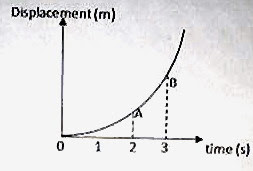## Pages

`“Life is like riding a bicycle.  To keep your balance you must keep moving.”–Albert Einstein`

## Monday, August 18, 2008

### AP Physics B & C- Multiple Choice Questions (for practice) on One Dimensional Motion

The essential points to be remembered in one dimensional and two dimensional motions were discussed in the post dated 14th August 2008. As promised, I give below some multiple choice questions on one dimensional motion. I’ll give you the solution in the next post.

The following questions will be useful for AP Physics B as well as C:(1) The adjoining figure shows the displacement-time graph of a particle in uniformly accelerated linear motion. At the points A and B (fig.) the graph is inclined at 30º and 60º respectively with the time axis. The acceleration of the particle in ms–2 is

(a) √2

(b) √3

(c) 3/√2

(d) 2/√3

(e) 1/√3

(2) A stone released from the top of a tower falls through half the height of the tower in 3/√2 seconds. The total time taken by the stone to reach the ground is

(a) 3 s

(b) 3.3 s

(c) 3.5 s

(d) 3.8 s

(e) 4 s

(3) A particle in one dimensional motion has initial velocity of 1 ms–1. The variation of its acceleration with time is represented by the semi circular curve shown in the adjoining figure. What is the velocity of the particle ( in ms–1) at the end of 8 seconds?(a) 2π

(b) 2π + 1

(c) 4π

(d) 4π + 1

(e) 1

(4) The bob of a simple pendulum is made to move along a semicircular path of radius 1 m. If the time taken to move along the semicircle is 1 s, the average velocity of the bob is

(a) π ms–1

(b) π/2 ms–1

(c) 1 ms–1

(d) 2 ms–1

(e) zero

(5) A stone is projected vertically upwards from point A (fig.) at the foot of a tower. It passes the top level B of the tower after 2 s. After another 2 s it again reaches the top level of the tower while returning from the top point C of its path. What is the height of the tower?(a) 98 m

(b) 55 m

(c) 40 m

(d) 22 m

(e) 10 m

The following Questions are for AP Physics C aspirants:

(1) The displacement x of a particle moving along a straight line is given by

x = 3t2 + 2t + 4 where x is in metre and t is in second.

If the particle could move for 3 seconds, what was its acceleration (in ms–2) at t = 2 s?

(a) 15

(b) 6

(c) 4

(d) 3

(e) 2

(2) A particle moving along the X-direction has its displacement x related to time t as

t = α x2 + β x where α and β are positive constants.

If the velocity of the particle at the instant t is v, its acceleration is

(a) α v2

(b) α v2

(c) 2 α v2

(d) 2α v3

(e) 2α v3

(3) A body falling freely under gravity covers half its total path in the last second of its fall. The total time taken by the body to cover the entire path is approximately

(a) 1.414 s

(b) 1.732 s

(c) 2.414 s

(d) 3.414 s

(e) 3.732 s

(4) The co-ordinates of a moving particle at time t are given by x = ax t2, y = ay t2 and z = az t2 where ax, ay, and az are constants. The magnitude of its velocity at time t is

(a) 2t (ax2 + ay2 + az2)1/2

(b) t (ax2 + ay2 + az2)1/2

(c) 2t (ax2 + ay2 + az2)

(d) t (ax2 + ay2 + az2)

(e) 2t (ax + ay + az)/3

Try to answer these questions. I’ll be back with the solution shortly.